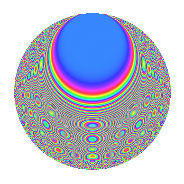# Properties

 Label 378.2.vLevel 378 Weight 2 Character orbit v Rep. character $$\chi_{378}(67,\cdot)$$ Character field $$\Q(\zeta_{9})$$ Dimension 144 Newforms 2 Sturm bound 144 Trace bound 7

# Learn more about

## Defining parameters

 Level: $$N$$ = $$378 = 2 \cdot 3^{3} \cdot 7$$ Weight: $$k$$ = $$2$$ Character orbit: $$[\chi]$$ = 378.v (of order $$9$$ and degree $$6$$) Character conductor: $$\operatorname{cond}(\chi)$$ = $$189$$ Character field: $$\Q(\zeta_{9})$$ Newforms: $$2$$ Sturm bound: $$144$$ Trace bound: $$7$$ Distinguishing $$T_p$$: $$5$$

## Dimensions

The following table gives the dimensions of various subspaces of $$M_{2}(378, [\chi])$$.

Total New Old
Modular forms 456 144 312
Cusp forms 408 144 264
Eisenstein series 48 0 48

## Trace form

 $$144q$$ $$\mathstrut +\mathstrut 6q^{6}$$ $$\mathstrut +\mathstrut 24q^{9}$$ $$\mathstrut +\mathstrut O(q^{10})$$ $$144q$$ $$\mathstrut +\mathstrut 6q^{6}$$ $$\mathstrut +\mathstrut 24q^{9}$$ $$\mathstrut +\mathstrut 24q^{11}$$ $$\mathstrut -\mathstrut 6q^{14}$$ $$\mathstrut -\mathstrut 12q^{15}$$ $$\mathstrut -\mathstrut 24q^{17}$$ $$\mathstrut -\mathstrut 30q^{21}$$ $$\mathstrut +\mathstrut 42q^{23}$$ $$\mathstrut -\mathstrut 36q^{26}$$ $$\mathstrut +\mathstrut 6q^{29}$$ $$\mathstrut +\mathstrut 18q^{30}$$ $$\mathstrut -\mathstrut 36q^{33}$$ $$\mathstrut -\mathstrut 36q^{35}$$ $$\mathstrut +\mathstrut 6q^{36}$$ $$\mathstrut -\mathstrut 72q^{39}$$ $$\mathstrut -\mathstrut 12q^{41}$$ $$\mathstrut +\mathstrut 48q^{42}$$ $$\mathstrut +\mathstrut 6q^{45}$$ $$\mathstrut +\mathstrut 18q^{47}$$ $$\mathstrut +\mathstrut 36q^{49}$$ $$\mathstrut +\mathstrut 12q^{50}$$ $$\mathstrut -\mathstrut 36q^{51}$$ $$\mathstrut -\mathstrut 30q^{53}$$ $$\mathstrut -\mathstrut 36q^{54}$$ $$\mathstrut -\mathstrut 6q^{56}$$ $$\mathstrut -\mathstrut 6q^{57}$$ $$\mathstrut -\mathstrut 60q^{59}$$ $$\mathstrut +\mathstrut 18q^{60}$$ $$\mathstrut +\mathstrut 18q^{61}$$ $$\mathstrut -\mathstrut 48q^{62}$$ $$\mathstrut -\mathstrut 60q^{63}$$ $$\mathstrut -\mathstrut 72q^{64}$$ $$\mathstrut +\mathstrut 54q^{65}$$ $$\mathstrut +\mathstrut 18q^{68}$$ $$\mathstrut -\mathstrut 60q^{69}$$ $$\mathstrut -\mathstrut 18q^{70}$$ $$\mathstrut +\mathstrut 24q^{71}$$ $$\mathstrut -\mathstrut 12q^{72}$$ $$\mathstrut +\mathstrut 72q^{73}$$ $$\mathstrut -\mathstrut 36q^{74}$$ $$\mathstrut +\mathstrut 12q^{75}$$ $$\mathstrut -\mathstrut 30q^{77}$$ $$\mathstrut +\mathstrut 36q^{78}$$ $$\mathstrut -\mathstrut 36q^{79}$$ $$\mathstrut -\mathstrut 12q^{80}$$ $$\mathstrut +\mathstrut 48q^{81}$$ $$\mathstrut -\mathstrut 6q^{84}$$ $$\mathstrut +\mathstrut 72q^{85}$$ $$\mathstrut -\mathstrut 24q^{86}$$ $$\mathstrut -\mathstrut 156q^{87}$$ $$\mathstrut -\mathstrut 72q^{89}$$ $$\mathstrut +\mathstrut 36q^{91}$$ $$\mathstrut +\mathstrut 42q^{92}$$ $$\mathstrut -\mathstrut 96q^{93}$$ $$\mathstrut -\mathstrut 36q^{94}$$ $$\mathstrut -\mathstrut 30q^{95}$$ $$\mathstrut -\mathstrut 24q^{98}$$ $$\mathstrut +\mathstrut 36q^{99}$$ $$\mathstrut +\mathstrut O(q^{100})$$

## Decomposition of $$S_{2}^{\mathrm{new}}(378, [\chi])$$ into irreducible Hecke orbits

Label Dim. $$A$$ Field CM Traces $q$-expansion
$$a_2$$ $$a_3$$ $$a_5$$ $$a_7$$
378.2.v.a $$72$$ $$3.018$$ None $$0$$ $$0$$ $$0$$ $$-6$$
378.2.v.b $$72$$ $$3.018$$ None $$0$$ $$0$$ $$0$$ $$6$$

## Decomposition of $$S_{2}^{\mathrm{old}}(378, [\chi])$$ into lower level spaces

$$S_{2}^{\mathrm{old}}(378, [\chi]) \cong$$ $$S_{2}^{\mathrm{new}}(189, [\chi])$$$$^{\oplus 2}$$